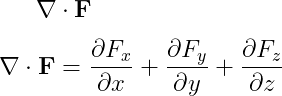# How to write a divergence operator(∇•F) in LaTeX?

Divergence operator is written in the form of the dot product of gradient operator() and vector.

div F= F (vector)

First, you can represent the divergence operator by arranging the individual symbols (nabla, dot, vector) one after the other.

\documentclass{article}
\begin{document}
$\mathbf{\nabla} \cdot \mathbf{F}$
$\mathbf{\nabla} \cdot \mathbf{F}=\frac{\partial F_{x}}{\partial x} + \frac{\partial F_{y}}{\partial y} + \frac{\partial F_{z}}{\partial z}$
\end{document}

Output :You notice that the output above is bold without the arrow symbol on F. And, this is the best practice with nabla(∇) symbol.

Second, you can represent the divergence operator with the help of physics package. This is because the \div command is present in this physics package. In which if you pass the vector as an argument, the divergence operator will return to you.

\documentclass{article}
\usepackage{physics}
\begin{document}
$\div$
$\div{\vb{F}}$
$\div(\vb{F_{1}+F_{2}})$
$\div[\vb{F_{x},F_{y},F_{z}}]$
\end{document}

Output :In latex, it is best practice for divergence operators to use physics packages rather than using each symbol individually.#### Md Jidan Mondal

LaTeX expert with over 10 years of experience in document preparation and typesetting. Specializes in creating professional documents, reports, and presentations using LaTeX.